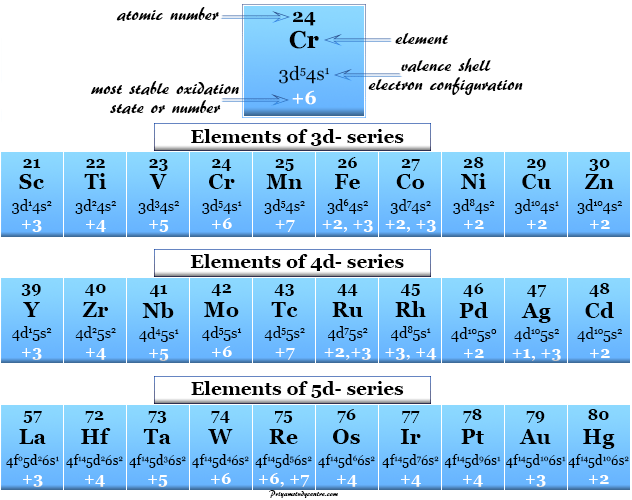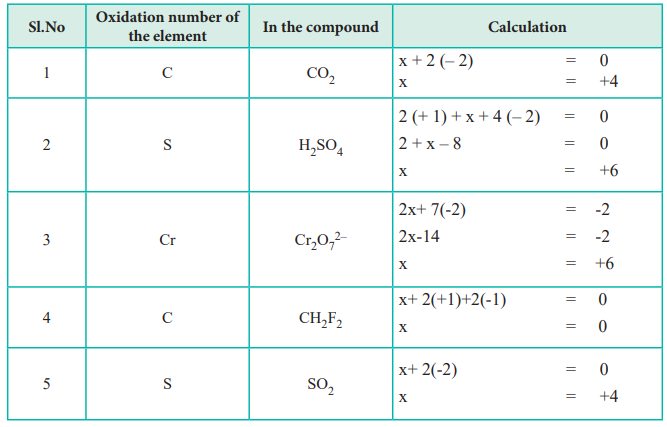# Rules for Oxidation Numbers Study Guide

Introduction

Have you seen that there is a layer of rust forming on the surface of any of the metal rods around you? Most often metal surfaces are painted, or a coating is applied to decrease rusting. Have you ever wondered why? It is because the metals get oxidized and develop rust when left untreated. Let’s learn about oxidation in this guide.Source

## RULES FOR ASSIGNING OXIDATION NUMBERS

Here are some of the rules for assigning oxidation numbers. They are as follows:

1. The pattern is that the cation is always written first in a formula, which is then followed by the anion.

2. The oxidation number of any free element always remains 0.

3. The oxidation number in the case of a monatomic ion is always equal to the value of the charge of the ion. For instance, the oxidation number of Na3- is 3-.

4. The expected oxidation number of hydrogen is plus 1. The oxidation number of hydrogen remains -1 in compounds, including elements that have less electronegativity than hydrogen, as in NaH.

5. The oxidation number of oxygen in the case of compounds is typically -2. Anomalies include OF2 because F turns out to be more electronegative than O, and BaO2, due to the construction of the peroxide ion, which can be expressed as (O-O)2-.

6. The oxidation number of elements in Group IA in a compound is +1.

7. The oxidation number of elements present in Group IIA in a compound is +2.

8. The oxidation number of elements present in Group VIIA in a compound is -1, except for the case when that element is conjoined with one having a higher value of electronegativity. The oxidation number of Cl turns out to be -1 in HCl, but this number becomes +1 in HOCl.

9. The sum of the value of oxidation numbers of all the present atoms in any neutral compound is 0.

10. Also, the sum of the value oxidation numbers in any given polyatomic ion is always equal to the value of the charge of the ion. For instance, the sum of the value of oxidation numbers for SO42- is -2.Source

## FAQs

1. What are the rules related to assigning oxidation numbers?

If you want to know how to assign oxidation numbers, you need to know some rules of oxidation numbers. Some of them are:

• The pattern is that the cation is expressed first in a formula, and then the anion is written.
• The oxidation number of any given free element always has to be 0.
• The oxidation number of a monatomic ion equals the value of the charge of the ion.

2. What is the easiest way to determine oxidation numbers?

The easiest way to determine the oxidation number is by dividing the total charge by two.

3. How do you find the oxidizing agent?

To find an oxidizing agent, you need to check the oxidation number of an atom that is before and after the chemical reaction. If the oxidation number is higher in the product, then it has lost electrons, and the substance went through oxidization. If the oxidation number is less, then it has gained electrons and was reduced.

We hope you enjoyed studying this lesson and learned something cool about Rules for Oxidation Numbers! Join our Discord community to get any questions you may have answered and to engage with other students just like you! Don’t forget to download our App to experience our fun VR classrooms – we promise it makes studying much more fun!😎

## SOURCES

1. Assigning Oxidation Numbers: https://www.ck12.org/c/chemistry/assigning-oxidation-numbers/lesson/Assigning-Oxidation-Numbers-CHEM/. Accessed 7th March 2022.
2. Rules for Assigning Oxidation Numbers: https://www.toppr.com/ask/question/write-the-rules-for-assigning-oxidation-number/. Accessed 7th March 2022.
]]>